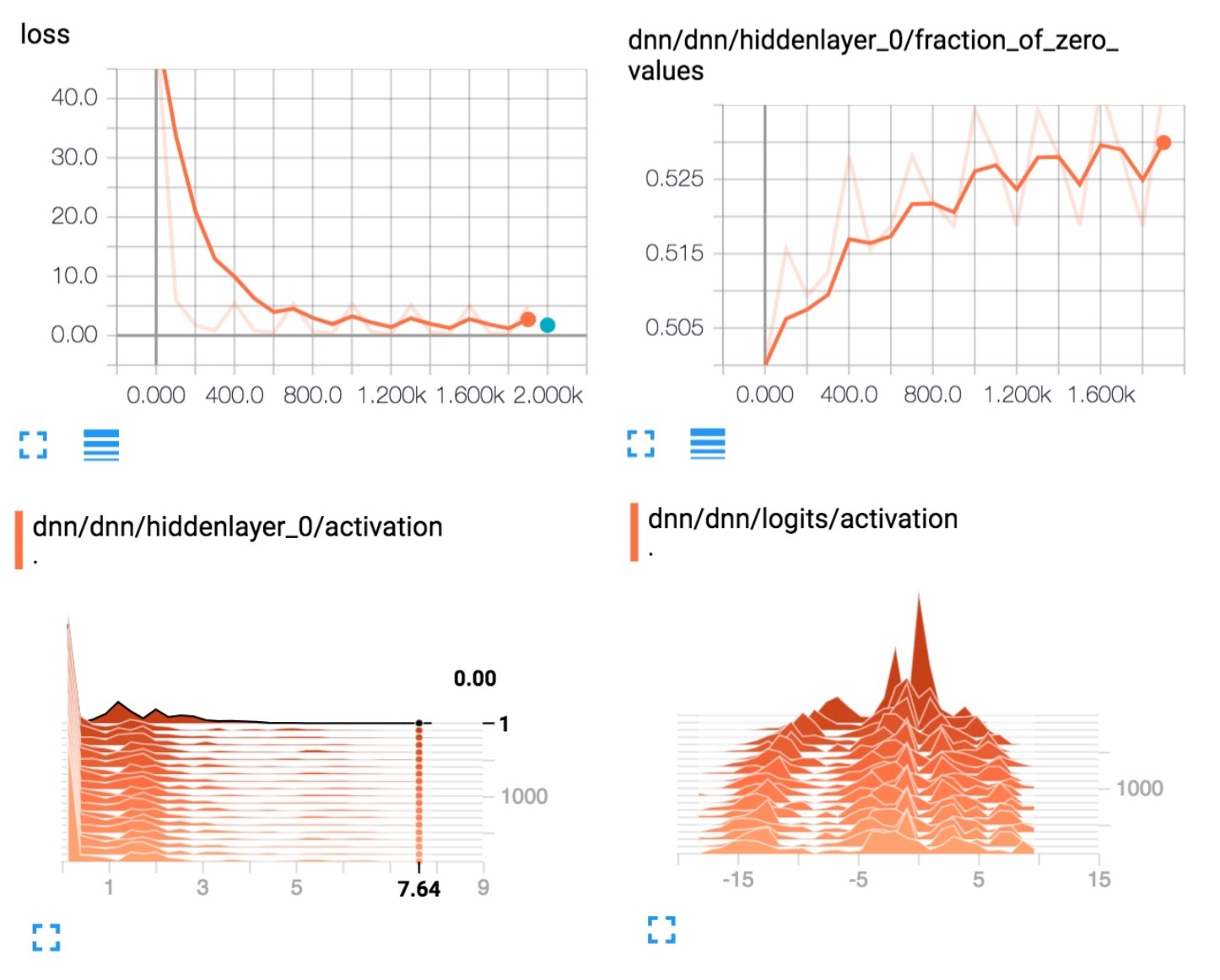V2EX = way to explore
V2EX 是一个关于分享和探索的地方

V2EX  ›  TensorFlow

# [TensorFlow 团队] TensorFlow 数据集和估算器介绍

fendouai_com · 2017-09-25 15:29:06 +08:00 · 4299 次点击

TensorFlow 1.3 引入了两个重要功能，您应当尝试一下： 数据集：一种创建输入管道（即，将数据读入您的程序）的全新方式。 估算器：一种创建 TensorFlow 模型的高级方式。估算器包括适用于常见机器学习任务的预制模型，不过，您也可以使用它们创建自己的自定义模型。 下面是它们在 TensorFlow 架构内的装配方式。结合使用这些估算器，可以轻松地创建 TensorFlow 模型和向模型提供数据：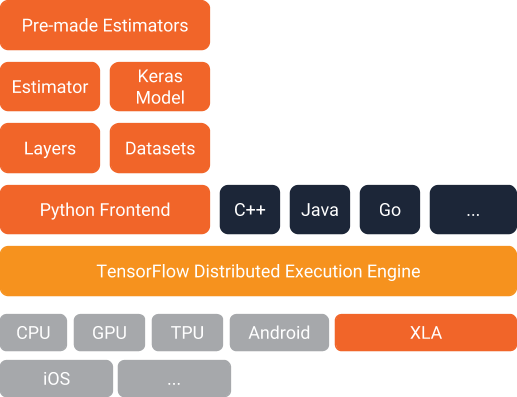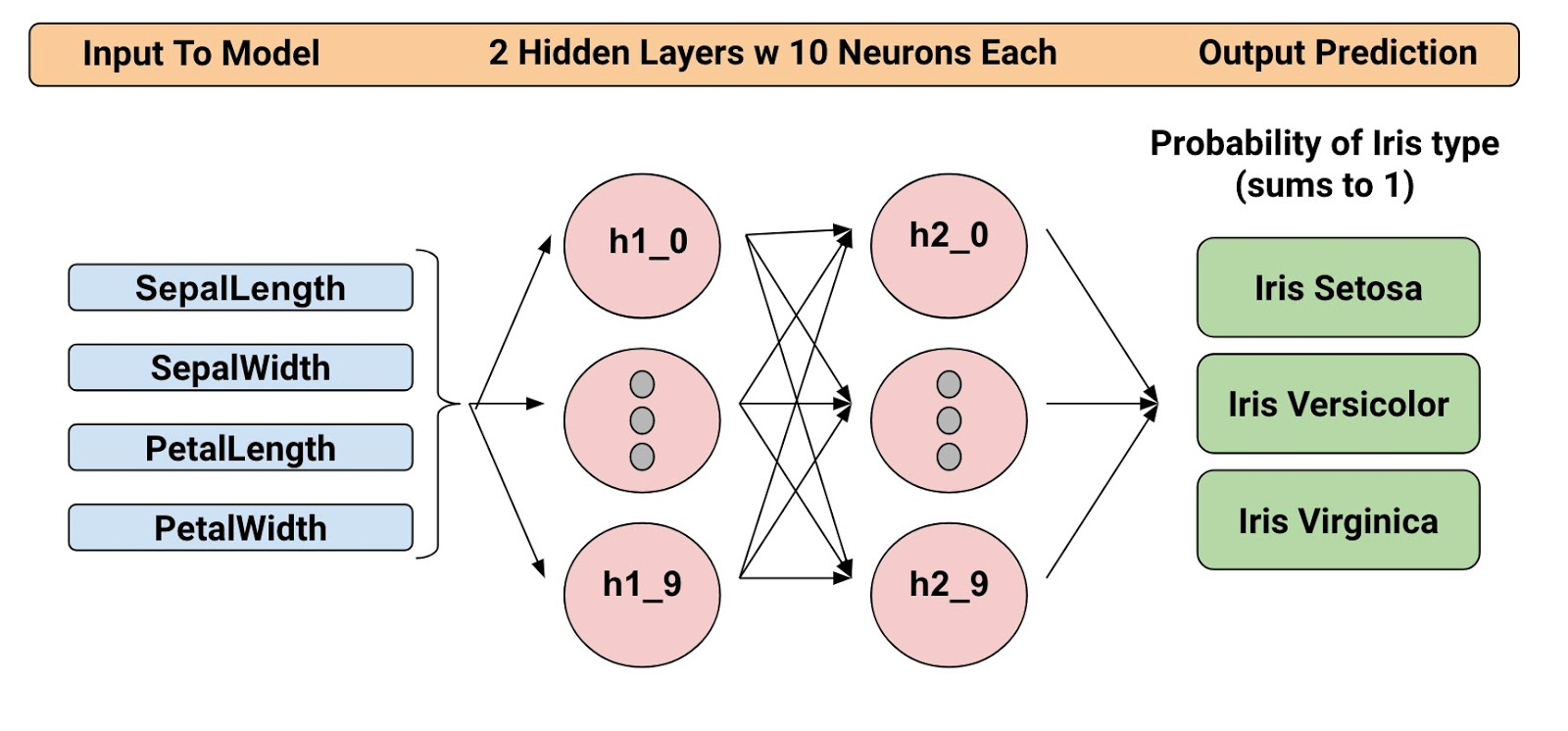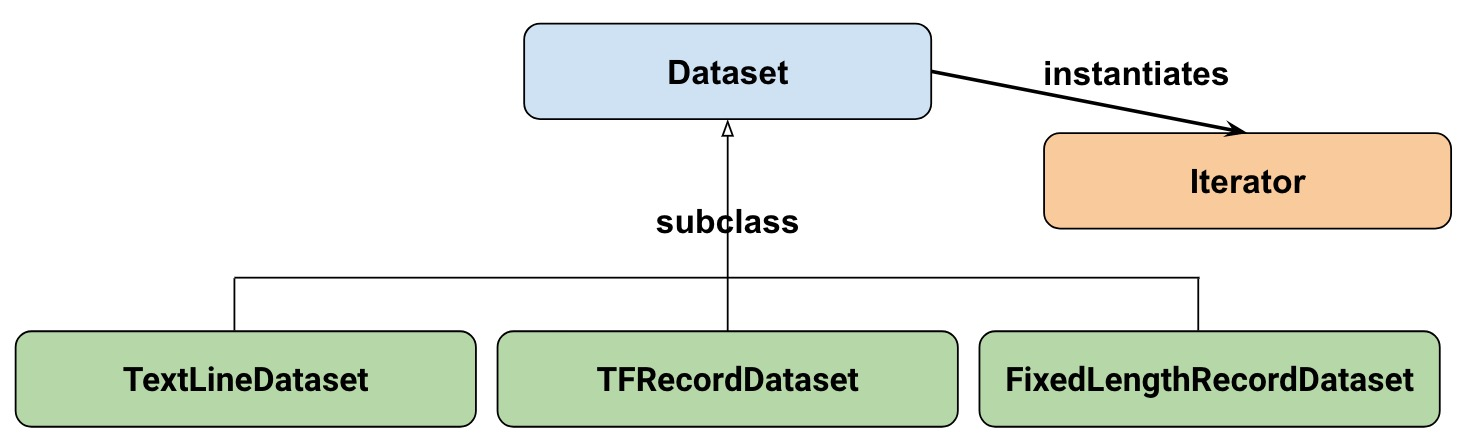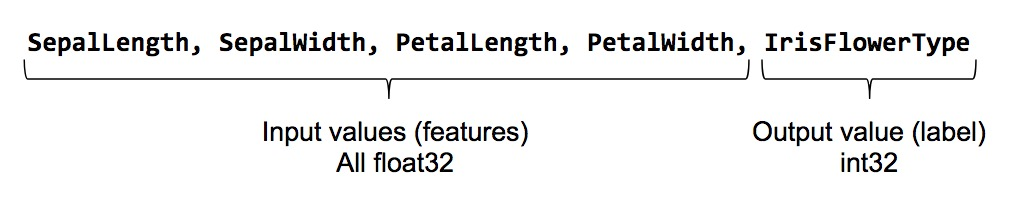``````feature_names = [
'SepalLength',
'SepalWidth',
'PetalLength',
'PetalWidth']

``````

``````
def input_fn():
...<code>...
return ({ 'SepalLength':[values], ..<etc>.., 'PetalWidth':[values] },
[IrisFlowerType])
``````

``````def my_input_fn(file_path, perform_shuffle=False, repeat_count=1):
def decode_csv(line):
parsed_line = tf.decode_csv(line, [[0.], [0.], [0.], [0.], ])
label = parsed_line[-1:] # Last element is the label
del parsed_line[-1] # Delete last element
features = parsed_line # Everything (but last element) are the features
d = dict(zip(feature_names, features)), label
return d

dataset = (tf.contrib.data.TextLineDataset(file_path) # Read text file
.map(decode_csv)) # Transform each elem by applying decode_csv fn
if perform_shuffle:
# Randomizes input using a window of 256 elements (read into memory)
dataset = dataset.shuffle(buffer_size=256)
dataset = dataset.repeat(repeat_count) # Repeats dataset this # times
dataset = dataset.batch(32)  # Batch size to use
iterator = dataset.make_one_shot_iterator()
batch_features, batch_labels = iterator.get_next()
return batch_features, batch_labels

``````

``````next_batch = my_input_fn(FILE, True) # Will return 32 random elements

# Now let's try it out, retrieving and printing one batch of data.
# Although this code looks strange, you don't need to understand
# the details.
with tf.Session() as sess:
first_batch = sess.run(next_batch)
print(first_batch)

# Output
({'SepalLength': array([ 5.4000001, ...<repeat to 32 elems>], dtype=float32),
'PetalWidth': array([ 0.40000001, ...<repeat to 32 elems>], dtype=float32),
...
},
[array([, ...<repeat to 32 elems>], dtype=int32) # Labels
)

``````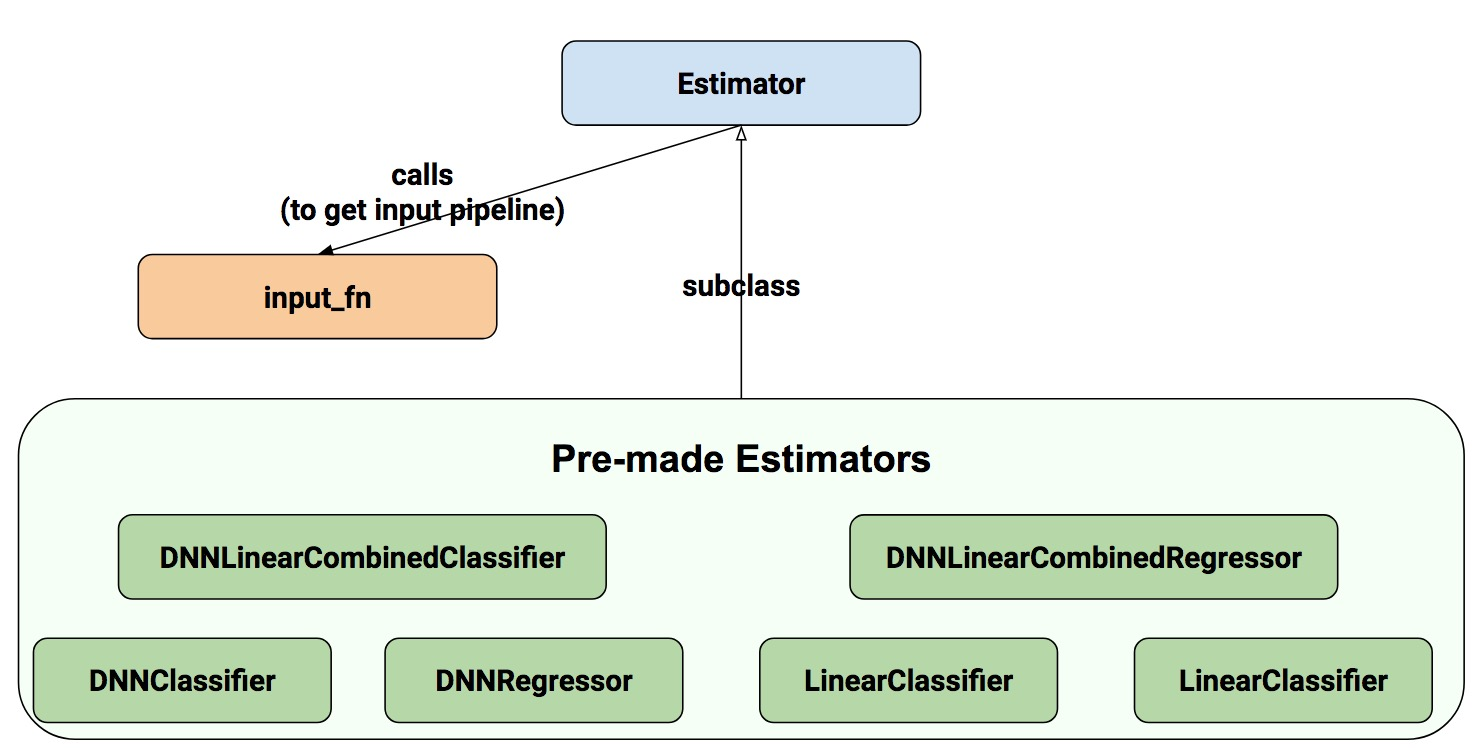``````# Create the feature_columns, which specifies the input to our model.
# All our input features are numeric, so use numeric_column for each one.
feature_columns = [tf.feature_column.numeric_column(k) for k in feature_names]

# Create a deep neural network regression classifier.
# Use the DNNClassifier pre-made estimator
classifier = tf.estimator.DNNClassifier(
feature_columns=feature_columns, # The input features to our model
hidden_units=[10, 10], # Two layers, each with 10 neurons
n_classes=3,
model_dir=PATH) # Path to where checkpoints etc are stored
``````

``````# Train our model, use the previously function my_input_fn
# Input to training is a file with training example
# Stop training after 8 iterations of train data (epochs)
classifier.train(
input_fn=lambda: my_input_fn(FILE_TRAIN, True, 8))
``````

``````# Evaluate our model using the examples contained in FILE_TEST
# Return value will contain evaluation_metrics such as: loss & average_loss
evaluate_result = estimator.evaluate(
input_fn=lambda: my_input_fn(FILE_TEST, False, 4)
print("Evaluation results")
for key in evaluate_result:
print("   {}, was: {}".format(key, evaluate_result[key]))
``````

``````# Predict the type of some Iris flowers.
# Let's predict the examples in FILE_TEST, repeat only once.
predict_results = classifier.predict(
input_fn=lambda: my_input_fn(FILE_TEST, False, 1))
print("Predictions on test file")
for prediction in predict_results:
# Will print the predicted class, i.e: 0, 1, or 2 if the prediction
# is Iris Sentosa, Vericolor, Virginica, respectively.
print prediction["class_ids"]
``````

``````# Let create a memory dataset for prediction.
# We've taken the first 3 examples in FILE_TEST.
prediction_input = [[5.9, 3.0, 4.2, 1.5],  # -> 1, Iris Versicolor
[6.9, 3.1, 5.4, 2.1],  # -> 2, Iris Virginica
[5.1, 3.3, 1.7, 0.5]]  # -> 0, Iris Sentosa
def new_input_fn():
def decode(x):
x = tf.split(x, 4) # Need to split into our 4 features
# When predicting, we don't need (or have) any labels
return dict(zip(feature_names, x)) # Then build a dict from them

# The from_tensor_slices function will use a memory structure as input
dataset = tf.contrib.data.Dataset.from_tensor_slices(prediction_input)
dataset = dataset.map(decode)
iterator = dataset.make_one_shot_iterator()
next_feature_batch = iterator.get_next()
return next_feature_batch, None # In prediction, we have no labels

# Predict all our prediction_input
predict_results = classifier.predict(input_fn=new_input_fn)

# Print results
print("Predictions on memory data")
for idx, prediction in enumerate(predict_results):
type = prediction["class_ids"] # Get the predicted class (index)
if type == 0:
print("I think: {}, is Iris Sentosa".format(prediction_input[idx]))
elif type == 1:
print("I think: {}, is Iris Versicolor".format(prediction_input[idx]))
else:
print("I think: {}, is Iris Virginica".format(prediction_input[idx])
``````

Dataset.from_tensor_slides() 面向可以装入内存的小数据集。按照与训练和评估时相同的方式使用 TextLineDataset 时，只要您的内存可以管理随机缓冲区和批次大小，您就可以处理任意大的文件。 拓展 使用像 DNNClassifier 一样的估算器可以提供很多值。除了易于使用外，预制估算器还提供内置的评估指标，并创建您可以在 TensorBoard 中看到的汇总。要查看此报告，请按照下面所示从您的命令行启动 TensorBoard：

``````# Replace PATH with the actual path passed as model_dir argument when the
# DNNRegressor estimator was created.
tensorboard --logdir=PATH
``````